Linear Regression and Correlation

# 72 Interpretation of Regression Coefficients: Elasticity and Logarithmic Transformation

As we have seen, the coefficient of an equation estimated using OLS regression analysis provides an estimate of the slope of a straight line that is assumed be the relationship between the dependent variable and at least one independent variable. From the calculus, the slope of the line is the first derivative and tells us the magnitude of the impact of a one unit change in the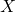variable upon the value of the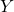variable measured in the units of thevariable. As we saw in the case of dummy variables, this can show up as a parallel shift in the estimated line or even a change in the slope of the line through an interactive variable. Here we wish to explore the concept of elasticity and how we can use a regression analysis to estimate the various elasticities in which economists have an interest.

The concept of elasticity is borrowed from engineering and physics where it is used to measure a material’s responsiveness to a force, typically a physical force such as a stretching/pulling force. It is from here that we get the term an “elastic” band. In economics, the force in question is some market force such as a change in price or income. Elasticity is measured as a percentage change/response in both engineering applications and in economics. The value of measuring in percentage terms is that the units of measurement do not play a role in the value of the measurement and thus allows direct comparison between elasticities. As an example, if the price of gasoline increased say 50 cents from an initial price of ?3.00 and generated a decline in monthly consumption for a consumer from 50 gallons to 48 gallons we calculate the elasticity to be 0.25. The price elasticity is the percentage change in quantity resulting from some percentage change in price. A 16 percent increase in price has generated only a 4 percent decrease in demand: 16% price change → 4% quantity change or .04/.16 = .25. This is called an inelastic demand meaning a small response to the price change. This comes about because there are few if any real substitutes for gasoline; perhaps public transportation, a bicycle or walking. Technically, of course, the percentage change in demand from a price increase is a decline in demand thus price elasticity is a negative number. The common convention, however, is to talk about elasticity as the absolute value of the number. Some goods have many substitutes: pears for apples for plums, for grapes, etc. etc. The elasticity for such goods is larger than one and are called elastic in demand. Here a small percentage change in price will induce a large percentage change in quantity demanded. The consumer will easily shift the demand to the close substitute.

While this discussion has been about price changes, any of the independent variables in a demand equation will have an associated elasticity. Thus, there is an income elasticity that measures the sensitivity of demand to changes in income: not much for the demand for food, but very sensitive for yachts. If the demand equation contains a term for substitute goods, say candy bars in a demand equation for cookies, then the responsiveness of demand for cookies from changes in prices of candy bars can be measured. This is called the cross-price elasticity of demand and to an extent can be thought of as brand loyalty from a marketing view. How responsive is the demand for Coca-Cola to changes in the price of Pepsi?

Now imagine the demand for a product that is very expensive. Again, the measure of elasticity is in percentage terms thus the elasticity can be directly compared to that for gasoline: an elasticity of 0.25 for gasoline conveys the same information as an elasticity of 0.25 for ?25,000 car. Both goods are considered by the consumer to have few substitutes and thus have inelastic demand curves, elasticities less than one.

The mathematical formulae for various elasticities are:

*** QuickLaTeX cannot compile formula:
\text{Price elasticity:}\phantom{\rule{0.1em}{0ex}}{\text{η}}_{\text{p}}=\frac{\left(\text{%∆Q}\right)}{\left(\text{%∆P}\right)}

*** Error message:
Package inputenc Error: Unicode character η (U+03B7)
File ended while scanning use of \frac .
Emergency stop.



Where η is the Greek small case letter eta used to designate elasticity. ∆ is read as “change”.

*** QuickLaTeX cannot compile formula:
\text{Income elasticity:}\phantom{\rule{0.1em}{0ex}}{\text{η}}_{\text{Y}}=\frac{\left(\text{%∆Q}\right)}{\left(\text{%∆Y}\right)}

*** Error message:
Package inputenc Error: Unicode character η (U+03B7)
File ended while scanning use of \frac .
Emergency stop.



Where Y is used as the symbol for income.

*** QuickLaTeX cannot compile formula:
\text{Cross-Price elasticity:}\phantom{\rule{0.1em}{0ex}}{\text{η}}_{\text{p1}}=\frac{\left(\text{%∆}{\text{Q}}_{1}\right)}{\left(\text{%∆}{\text{P}}_{2}\right)}

*** Error message:
Package inputenc Error: Unicode character η (U+03B7)
File ended while scanning use of \frac .
Emergency stop.



Where P2 is the price of the substitute good.

Examining closer the price elasticity we can write the formula as:

*** QuickLaTeX cannot compile formula:
{\text{η}}_{\text{p}}=\frac{\left(\text{%∆Q}\right)}{\left(\text{%∆P}\right)}=\frac{\text{dQ}}{\text{dP}}\left(\frac{\text{P}}{\text{Q}}\right)=\text{b}\left(\frac{\text{P}}{\text{Q}}\right)

*** Error message:
Package inputenc Error: Unicode character η (U+03B7)
File ended while scanning use of \frac .
Emergency stop.



Where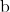is the estimated coefficient for price in the OLS regression.

The first form of the equation demonstrates the principle that elasticities are measured in percentage terms. Of course, the ordinary least squares coefficients provide an estimate of the impact of a unit change in the independent variable, X, on the dependent variable measured in units of Y. These coefficients are not elasticities, however, and are shown in the second way of writing the formula for elasticity as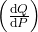, the derivative of the estimated demand function which is simply the slope of the regression line. Multiplying the slope times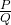provides an elasticity measured in percentage terms.

Along a straight-line demand curve the percentage change, thus elasticity, changes continuously as the scale changes, while the slope, the estimated regression coefficient, remains constant. Going back to the demand for gasoline. A change in price from ?3.00 to ?3.50 was a 16 percent increase in price. If the beginning price were ?5.00 then the same 50¢ increase would be only a 10 percent increase generating a different elasticity. Every straight-line demand curve has a range of elasticities starting at the top left, high prices, with large elasticity numbers, elastic demand, and decreasing as one goes down the demand curve, inelastic demand.

In order to provide a meaningful estimate of the elasticity of demand the convention is to estimate the elasticity at the point of means. Remember that all OLS regression lines will go through the point of means. At this point is the greatest weight of the data used to estimate the coefficient. The formula to estimate an elasticity when an OLS demand curve has been estimated becomes: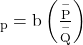Where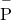and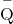are the mean values of these data used to estimate, the price coefficient.

The same method can be used to estimate the other elasticities for the demand function by using the appropriate mean values of the other variables; income and price of substitute goods for example.

### Logarithmic Transformation of the Data

Ordinary least squares estimates typically assume that the population relationship among the variables is linear thus of the form presented in The Regression Equation. In this form the interpretation of the coefficients is as discussed above; quite simply the coefficient provides an estimate of the impact of a one unit change in X on Y measured in units of Y. It does not matter just where along the line one wishes to make the measurement because it is a straight line with a constant slope thus constant estimated level of impact per unit change. It may be, however, that the analyst wishes to estimate not the simple unit measured impact on the Y variable, but the magnitude of the percentage impact on Y of a one unit change in the X variable. Such a case might be how a unit change in experience, say one year, effects not the absolute amount of a worker’s wage, but the percentage impact on the worker’s wage. Alternatively, it may be that the question asked is the unit measured impact on Y of a specific percentage increase in X. An example may be “by how many dollars will sales increase if the firm spends X percent more on advertising?” The third possibility is the case of elasticity discussed above. Here we are interested in the percentage impact on quantity demanded for a given percentage change in price, or income or perhaps the price of a substitute good. All three of these cases can be estimated by transforming the data to logarithms before running the regression. The resulting coefficients will then provide a percentage change measurement of the relevant variable.

To summarize, there are four cases:

1. Unit ∆X → Unit ∆Y (Standard OLS case)
2. Unit ∆X → %∆Y
3. %∆X → Unit ∆Y
4. %∆X → %∆Y (elasticity case)

Case 1: The ordinary least squares case begins with the linear model developed above: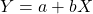where the coefficient of the independent variable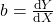is the slope of a straight line and thus measures the impact of a unit change in X on Y measured in units of Y.

Case 2: The underlying estimated equation is: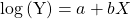The equation is estimated by converting the Y values to logarithms and using OLS techniques to estimate the coefficient of the X variable, b. This is called a semi-log estimation. Again, differentiating both sides of the equation allows us to develop the interpretation of the X coefficient b: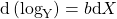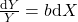Multiply by 100 to covert to percentages and rearranging terms gives:

*** QuickLaTeX cannot compile formula:
100b=\frac{\text{%∆Y}}{\text{Unit ∆X}}

*** Error message:
File ended while scanning use of \frac .
Emergency stop.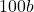is thus the percentage change in Y resulting from a unit change in X.

Case 3: In this case the question is “what is the unit change in Y resulting from a percentage change in X?” What is the dollar loss in revenues of a five percent increase in price or what is the total dollar cost impact of a five percent increase in labor costs? The estimated equation for this case would be: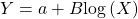Here the calculus differential of the estimated equation is: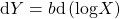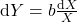Divide by 100 to get percentage and rearranging terms gives:

*** QuickLaTeX cannot compile formula:
\frac{b}{100}=\frac{\text{d}Y}{100\frac{\text{d}X}{X}}=\frac{\text{Unit ∆Y}}{\text{%∆X}}

*** Error message:
File ended while scanning use of \frac .
Emergency stop.



Therefore,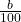is the increase in Y measured in units from a one percent increase in X.

Case 4: This is the elasticity case where both the dependent and independent variables are converted to logs before the OLS estimation. This is known as the log-log case or double log case, and provides us with direct estimates of the elasticities of the independent variables. The estimated equation is: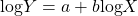Differentiating we have: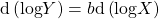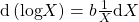thus: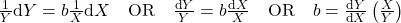and

*** QuickLaTeX cannot compile formula:
b=\frac{\text{%∆Y}}{\text{%∆X}}

*** Error message:
File ended while scanning use of \frac .
Emergency stop.



our definition of elasticity. We conclude that we can directly estimate the elasticity of a variable through double log transformation of the data. The estimated coefficient is the elasticity. It is common to use double log transformation of all variables in the estimation of demand functions to get estimates of all the various elasticities of the demand curve.

In a linear regression, why do we need to be concerned with the range of the independent (X) variable?

The precision of the estimate of the Y variable depends on the range of the independent (X) variable explored. If we explore a very small range of the X variable, we won’t be able to make much use of the regression. Also, extrapolation is not recommended.

Suppose one collected the following information where X is diameter of tree trunk and Y is tree height.

 X Y 4 8 2 4 8 18 6 22 10 30 6 8

Regression equation: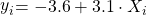What is your estimate of the average height of all trees having a trunk diameter of 7 inches?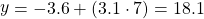The manufacturers of a chemical used in flea collars claim that under standard test conditions each additional unit of the chemical will bring about a reduction of 5 fleas (i.e. where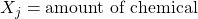and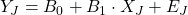,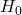: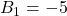Suppose that a test has been conducted and results from a computer include:

Intercept = 60

Slope = −4

Standard error of the regression coefficient = 1.0

Degrees of Freedom for Error = 2000

95% Confidence Interval for the slope −2.04, −5.96

Is this evidence consistent with the claim that the number of fleas is reduced at a rate of 5 fleas per unit chemical?

Most simply, since −5 is included in the confidence interval for the slope, we can conclude that the evidence is consistent with the claim at the 95% confidence level.

Using a t test::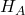: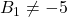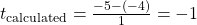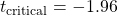Since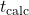<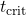we retain the null hypothesis that.